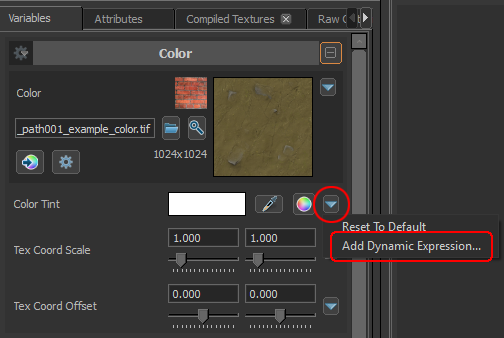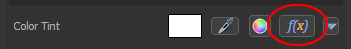# Dynamic Material Expressions

Dynamic material expressions can be used to procedurally animate a material parameter over time or to drive it by values supplied by game code in order for the material to represent certain game state.

## Adding a new dynamic expression in the Material Editor

1. Click the little triangle button to the right of a material parameter:2. In the dialog that pops open, enter your expression. A simple example would be
```0.5 * sin( 2*time() ) + 0.5
```
to see a parameter cycling smoothly between 0 and 1.
3. To edit an existing dynamic expression on a material parameter, click on the "f(x)" button.4. To remove a dynamic expression from a material parameter open the edit dialog and clear the edit field.

## Dynamic expressions on texture parameters vs other parameters

It is important to understand the difference between texture parameters and other parameters such as "Color Tint" and scalar parameters with UI sliders: The "Color Tint" parameter feeds a numeric value to the shader that can be computed dynamically with a material expression such as the example in the section above. A texture parameter feeds a texture map to the shader, so if you try to add an expression that computes a color value on a texture parameter it won't work.

Dynamic expressions on texture parameters are for advanced use only: These expressions have to reference an attribute supplied by game code that points to a texture.

## Dynamic Expression Reference

Material expressions look very similar to HLSL code. Expressions can have one of two basic structures:

A simple inline expression that's just one math expression. Examples:

```sin(SomeAttribute)
```
```10 + sqrt( 2 ) * frac( time() )
```

Expressions can also be composed of multiple intermediate expressions -- each of which computes a temporary value -- followed by a return expression that uses the intermediate values. Example:

```tempVar1 = sin(SomeAttribute);
tempVar2 = exists(SomeOtherAttribute) ? float4( 1, 2, 3, 4 ) : float4( 5, 6, 7, 8 );
tempVar3 = cos(tempVar1)
return tempVar1 + dot4( tempVar2, SomeThirdAttribute.xyzz ) * tempVar3;
```

### Identifiers

Identifiers in expressions can refer either to intermediate values stored in local variables (e.g. `tempVar1` in the example above) or to attributes supplied by game code (e.g. `SomeAttribute` in the example above). If an identifier isn't a local variable it is assumed to be supplied by game code.

If an expression relies on a value supplied by game code but the code doesn't provide it, expression evaluation will silently fail and the value of the parameter with the expression will fall back to the value set for the parameter in the material.

### Data Types

The internal type for computations is float4, everything gets converted to a four-component float internally by smearing the last specified component into the remaining ones.

Examples:

• 3.5 becomes (3.5, 3.5, 3.5, 3.5)
• float2( 1, 2 ) becomes (1, 2, 2, 2)
• float3( 1, 2, 3 ) becomes (1, 2, 3, 3)
• vSomeVector.xyz becomes vSomeVector.xyzz
• vVec3.zx becomes vVec3.zxxx

Any scalar function is applied to all four components of the input vectors. Any function that returns a scalar result will output the same result to all four components.

### Operators

Supported operators in order of precedence:

1. function call
2. ()
3. . (for swizzles, e.g. .xyxy)
4.  ! - (- meaning negate)
5. * / % (muliply, divide, modulo)
7. < > <= >= (comparisons)
8. == != (equality, inequality)
9. && (logical and. Lazy evaluation like in C.)
10. || (logical or. Lazy evaluation like in C.)
11.  ?: (conditional. Lazy evaluation: Only the subexpression indicated by the predicate is evaluated.)
12. = (assignment)

### Builtin Functions

All functions take float4() as parameters. All scalar operations will be applied to all four components of the input. For example sin(vSomeVector) will compute the output vector (sin(vSomeVector.x),sin(vSomeVector.y),sin(vSomeVector.z),sin(vSomeVector.w))

• `exists(x)` - Returns 1 if the attribute named x exists, 0 otherwise
• `sin(x)`
• `cos(x)`
• `tan(x)`
• `frac(x)` - Fractional part of each floating point value
• `floor(x)` - Returns the largest int that's smaller than x
• `ceil(x)` - Returns the smallest int that's larger than x
• `saturate(x)` - Clamps x between 0 and 1
• `clamp(x, min, max)` - Clamps x between min and max
• `lerp(a, b, t)` - Linearly interpolates t between a and b (returns a for t=0 and b for t=1)
• `dot4(v0, v1)` - Four-component dot product
• `dot3(v0, v1)` - Three-componend dot product
• `dot2(v0, v1)` - Two-component dot product
• `log(x)`
• `log2(x)`
• `log10(x)`
• `exp(x)`
• `exp2(x)`
• `sqrt(x)`
• `rsqrt(x)` - 1/sqrt(x)
• `sqr(x)` - Returns x*x
• `sign(x)`
• `abs(x)`
• `pow(x, y)`
• `step(a, b)` - If ( a >= b ) return 1; else return 0;
• `smoothstep( a, b, t )` - Return 0 for t==a, 1 for t==b, smoothly interpolate using a cubic polynomial for 0<t<1
• `float4(v0, v1, v2, v3)` - Returns (v0.x, v1.x, v2.x, v3.x)
• `float3(v0, v1, v2)` - Returns (v0.x, v1.x, v2.x, v2.x)
• `float2(v0, v1)` - Returns (v0.x, v1.x, v1.x, v1.x)
• `time()` - Returns the current time
• `min(v0, v1)`
• `max(v0, v1)`
• `SrgbLinearToGamma(x)`
• `SrgbGammaToLinear(x)`
• `random(xMin, xMax)` - Returns a random value between xMin.x and xMax.x
• `normalize(v0)` - Returns the normalized 3-vector in the xyz components. w component remains unchanged.
• `length(v0)` - Returns the length of 3-vector in xyz.